Get instant live expert help with Excel or Google Sheets“My Excelchat expert helped me in less than 20 minutes, saving me what would have been 5 hours of work!”

#### Post your problem and you’ll get Expert help in seconds.

Your message must be at least 40 characters
Our professional Expert are available now. Your privacy is guaranteed.

# Indirect Named Range Different Sheet

Excel allows us the ability to reference another sheet based on another cell’s value. This step by step tutorial will assist all levels of Excel users in using the INDIRECT function to reference another sheet.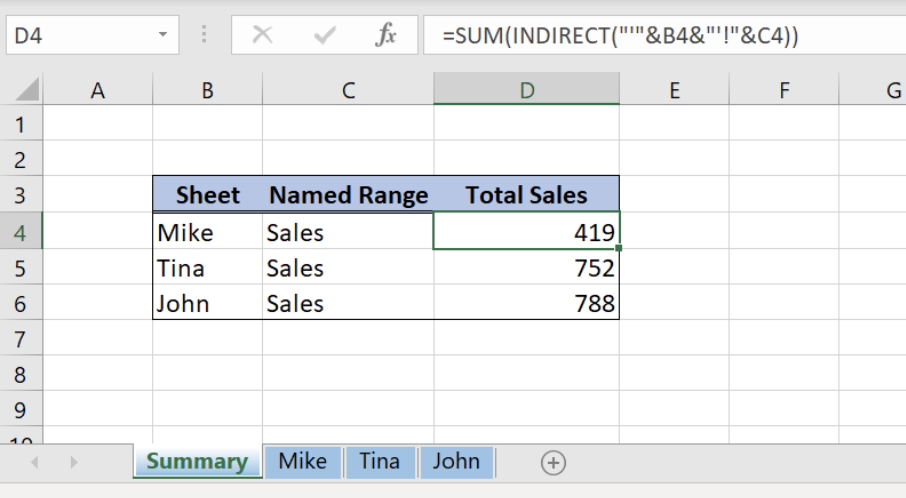Figure 1. Final result

## Syntax of the INDIRECT and SUM formula

The generic formula for summing cells from the specific sheet and cell range:

`=SUM(INDIRECT("'"&Sheet&"'!"&Named range))`

The components of the formula are:Q

• SUM – this Excel function will summarise the data from the specific sheet and the cell range
• INDIRECT – by this Excel function we can reference the specific sheet and the cell range where we want to summarise the data
• Named range – a range of values which we want to sum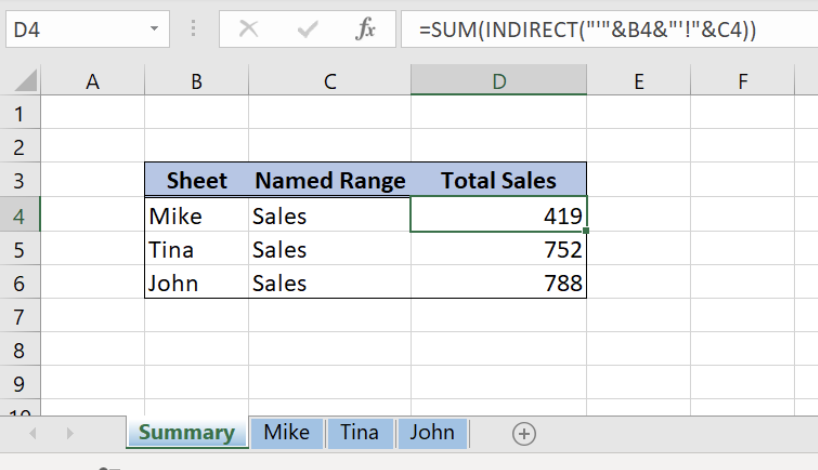Figure 1. Indirect named range different sheet

We will now look at the example to explain in detail how to reference the named range to another sheet. Let’s start with examining the structure of the data that we will use.

In the “Summary” sheet in the column “Total Sales” we want to show total sales per each employee (Figure 1). Therefore, we created three sheets with employees’ names, “Mike”, ”Tina” and “John”. Thus, all employees’ sheets contain data about Sales per product type (Figure 2).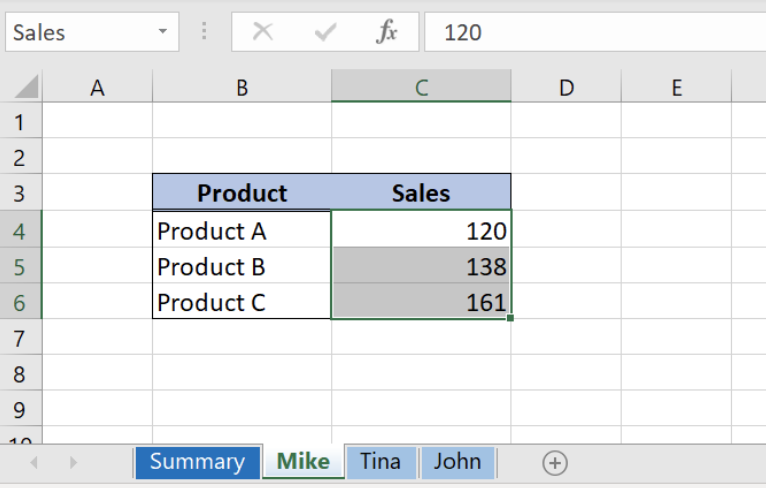Figure 2. Sales data per product and employee

## Calculate Total Sales Using SUM and INDIRECT

We want to calculate total sales per each employee by using a named range “Sales”. Sales data per employee are in separate sheets “Mike”, “Tina” and “John”. We can do this with the combination of SUM and INDIRECT function.

The formula in the Summary sheet looks like:

`=SUM(INDIRECT("'"&B4&"'!"&C4))`

The formula summarises the data from the sheet defined in the column “Sheet” and the cell range in the column “Named Range”. Since the named range is the part of our formula, we will have to explain how can we create them in Excel.

## Create Named Ranges

Follow these steps to create named ranges:

• In Formula tab click on the Name Manager
• In popup screen select New
• A new window will appear and we will have to define “Name”, “Scope” and “Refers to”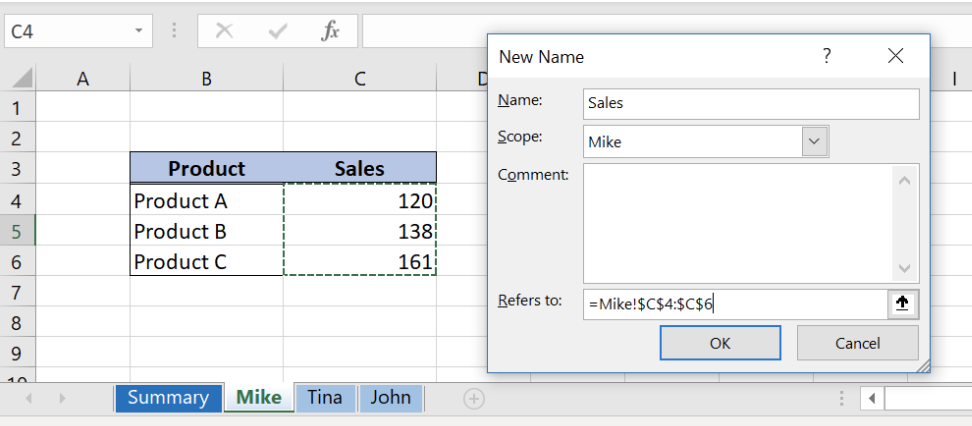Figure 3. How to create named range in Name Manager

• Name of the range will be “Sales”, Scope will be sheet “Mike”, while Refers to will be Sales data in the table on the left-hand side that we want to summarise
• Just click OK and we created a named range “Sales”
• In order to create the same named range but for different employees, just repeat steps above and change Scope to “Tina” and “John” sheet.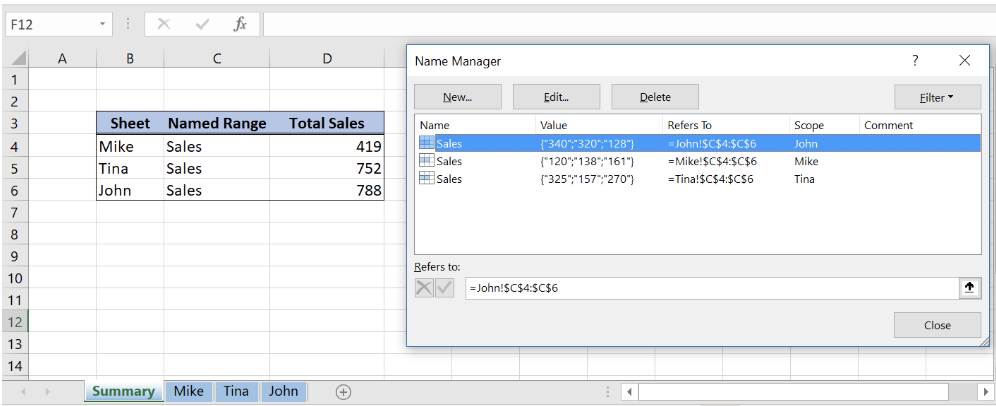Figure 4. Named ranges for sales data per employee

• As a result, in Figure 4, three named ranges referencing to sales data per each employee in sheets “Mike”, “Tina” and “John”

## Calculate Total Sales per Employee

Finally, we can go back to our formula in “Summary” sheet where we want to calculate the total sales per employee.Figure 5. Total Sales per employee

The formula for Total Sales looks like:

`=SUM(INDIRECT("'"&B4&"'!"&C4))`

Let’s now explain all formula parts:

• SUM – summarise the data from the named range in the column “Named Range” and from the Sheet in the column “Sheet”
• INDIRECT– we can use this formula to refer to specific Sheet and cell range defined by named range

The result from the INDIRECT formula will look like:

`=SUM(INDIRECT("'Mike'!Sales"))`

As we can see INDIRECT function reference to Sheet “Mike” and named range “Sales”. Finally, SUM function will summarise data from “Sales” named range and sheet “Mike” giving formula result 419.

## Notes

• Use single quotations for sheet name if we are not sure that sheet name contains spaces
• Please note that we put absolute cell reference (\$ in front of the cells)
• We can refer to a named range without INDIRECT function using the formula:

`=SUM('Mike'!Sales)`

Most of the time, the problem we will need to solve will be more complex than a simple application of a formula or function. If you want to save hours of research and frustration, try our live Excelchat service! Our Excel Experts are available 24/7 to answer any Excel question you may have. We guarantee a connection within 30 seconds and a customized solution within 20 minutes.

### Did this post not answer your question? Get a solution from connecting with the expert.Another blog reader asked this question today on Excelchat:
Solution examplesHello, I need help regarding indirect formula of excel. 1. What is the purpose of using this formula? 2. If we use this formula with sum formula, then why we are using this with sum formula, we can also perform addition from different sheets without indirect formula ?
Solved by O. D. in 21 minsI need a formula to pop at the upper left corner of a spreadsheet. If I enter the month "January," I want the column number sum of January =SUM(AB11:AB75) from another section on the same excel page to pop right below the "January" cell, and not display the formula expression, but see the \$100.
Solved by T. Q. in 40 minsCan't add (SUM) in imported numbers from bank account
Solved by F. C. in 40 minsI need a formula to combine D2 to D100 to add together a column of numbers, then take away the same amount on the same row when column E is filled. i.e. column D is a price of an item, so the formula must calculate the total, then when the item is sold an 'a' is marked next to the item in column E, the formula then must deduct this amount from the total
Solved by X. W. in 20 minsI would like to have a diagram in a new sheet, where the horizontal axis is the days, as they are in column DX. Each day shall show the sum of all unique leads of that day, and I would like to be able to check via a box of checkboxes, which facilities are shown, the facilities are in column BC.
Solved by I. A. in 45 mins## Subscribe to Excelchat.coAnother blog reader asked this question today on Excelchat: